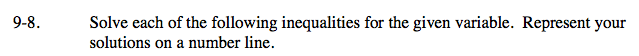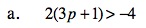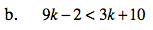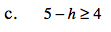### Home > CAAC > Chapter 9 > Lesson 9.1.1 > Problem9-8

9-8.Solve the equation 2(3p +1) = −4 to find the boundary point.

Test values on both sides of the boundary point (p = −1) to determine the solution region.

2(3(−2) + 1) > −4 2(3(0) +1) > −4
2(−5) > −4 2(1) > −4
−10 > −4 2 > −4
False TrueUse the eTool below to represent your solutions on a number line.
Click the link at right for the full version of the eTool: AC 9-8 HW eTool